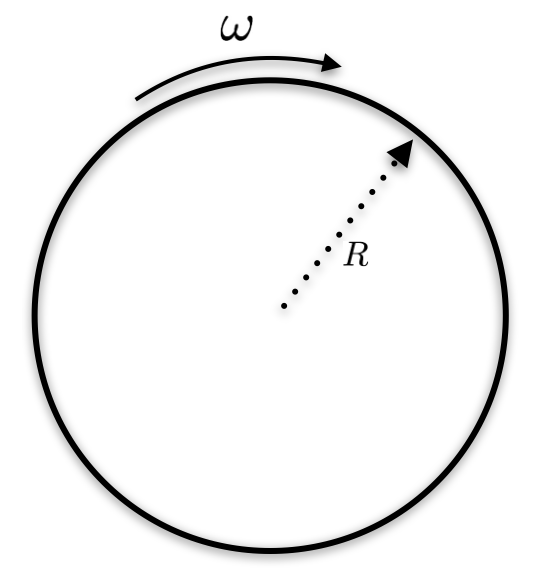# Relativistic Rotating DiskA disk with radius $R=1 \space m$ is rotating with a large angular velocity of $\omega =2.5\cdot { 10 }^{ 8 }\space s^{ -1 }$. According to relativistic kinematics, what is the ratio of the disk's circumference to its radius, as observed in an inertial frame where the disk does not translate?

Bonus Thought: According to elementary geometry, the answer is $2 \pi$. Is it? If not, what's going on here?

×